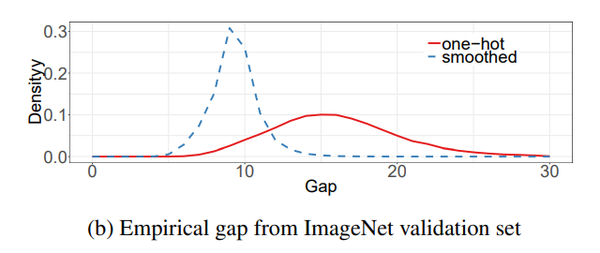# 浅谈LabelSmooth的两种实现以及推导_蒋

【GiantPandaCV导语】因为最近跑VIT的实验，所以有用到timm的一些配置，在mixup的实现里面发现labelsmooth的实现是按照最基本的方法来的，与很多pytorch的实现略有不同，所以简单做了一个推导。

## 一、交叉熵损失(CrossEntropyLoss)¶

loss = -\sum_{i=1}^{n} y_{i}log(x _{i}) \\

• log_softmax

\sum_{i=1}^{n}log(\frac{exp(x_{i})}{\sum_{i=1}^{n}(exp(x_{i}))}) \\
• NLLloss

L(\theta) = \sum_{i}^nlog(P(x_{i};\theta))\\

L(\theta) = -\sum_{i}^nlog(P(x_{i};\theta))\\

L(\theta) = -log(P(x_{i};\theta))\\

## 二、LabelSmooth¶y(i) = \left\{ \begin{array}{**lr**} \frac{\varepsilon}{n}, & i \ne target\\ 1 - \varepsilon + \frac{\varepsilon}{n} & i = target\\ \end{array} \right. \\

Loss = -\sum_i^{n}y(i)log(p(x_{i}))\\

## 三、公式推导¶

# labelsmooth
import torch
import torch.nn as nn
import torch.nn.functional as F

class LabelSmoothingCrossEntropy(nn.Module):
"""
NLL loss with label smoothing.
"""
def __init__(self, smoothing=0.1):
"""
Constructor for the LabelSmoothing module.
:param smoothing: label smoothing factor
"""
super(LabelSmoothingCrossEntropy, self).__init__()
assert smoothing < 1.0
self.smoothing = smoothing
self.confidence = 1. - smoothing

def forward(self, x, target):
logprobs = F.log_softmax(x, dim=-1)
nll_loss = -logprobs.gather(dim=-1, index=target.unsqueeze(1))
nll_loss = nll_loss.squeeze(1)
smooth_loss = -logprobs.mean(dim=-1)
loss = self.confidence * nll_loss + self.smoothing * smooth_loss
return loss.mean()


\begin{align*} Loss &= -\sum_{i=1}^n y(x_i)log(p(x_{i}))*(1-\varepsilon) + -\frac{1}{n}\sum_{i=1}^{n}log(x_i)*\varepsilon\\ & = -\sum_{i=1}^{n}y(x_i)log(p(x_{i}))(1-\varepsilon)+ -\frac{1}{n}(\varepsilon*log(p(x_1)) + \varepsilon*log(p(x_2)) + ... + \varepsilon*log(p(x_n)) ) \end{align*}\\

\begin{align*} Loss &= -（1 -\varepsilon）* log(p(x_k))+ -\frac{1}{n}(\varepsilon*log(p(x_1)) +...+ \varepsilon*log(p(x_k-1)) + \varepsilon*log(p(x_k+1)) +...+ \varepsilon*log(p(x_n)) ) \\ \end{align*}\\

\begin{align*} Loss &= -（1+\frac{\varepsilon}{n} -\varepsilon）* log(p(x_k))+ -\frac{1}{n}(\varepsilon*log(p(x_1)) + \varepsilon*log(p(x_2)) + ... + \varepsilon*log(p(x_n)) ) \\ &= -（1+\frac{\varepsilon}{n} -\varepsilon）* log(p(x_k)) + -\frac{\varepsilon}{n}*(log(p(x_1))+...+log(p(x_{k-1})) + log(p(x_k+1)) + ... + log(p(x_n)))\\ &= -（1+\frac{\varepsilon}{n} -\varepsilon）* log(p(x_k)) + \frac{\varepsilon}{n} \sum_{i=1, i\ne k}^n log(p(x_i)) \end{align*}\\

Loss=\left[ log(p(x_1)), ...,log(p(x_k)),..., log(p(x_n))\right]\odot \left[ \frac{\varepsilon}{n}, ..., (1 - \varepsilon +\frac{\varepsilon}{n}）,..., \frac{\varepsilon}{n} \right]\\

Loss= -\sum_i^{n}y(x_{i})log(p(x_{i}))\\

def one_hot(x, num_classes, on_value=1., off_value=0., device='cuda'):
x = x.long().view(-1, 1)

def mixup_target(target, num_classes, lam=1., smoothing=0.0, device='cuda'):
off_value = smoothing / num_classes
on_value = 1. - smoothing + off_value
y1 = one_hot(target, num_classes, on_value=on_value, off_value=off_value, device=device)
y2 = one_hot(target.flip(0), num_classes, on_value=on_value, off_value=off_value, device=device)
return y1 * lam + y2 * (1. - lam)


## 四，总结¶

LabelSmooth可以用来标签平滑，从公示推导方面来讲，也可以充当正则的作用，尤其是针对难分类别的情况下，效果会表现更好一些。